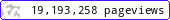•••••MadAsMaths.com :: Maths Booklets :: Statistics

STATISTICS

These booklets are suitable for

• students taking statistical modules in A Level Mathematics or A Level Further Mathematics.

• introduction/revision/practice to statistical topics, for undergraduates in various degrees/diplomas or mathematics degrees.

 binomial_distribution.pdfcombinations_and_permutations.pdfcontingency_tables.pdfcorrelation_and_regression.pdfdata_analysis.pdfdata_analysis_exam_questions.pdfdiscrete_random_variables.pdfdistributional_approximations.pdfgeometric_distribution.pdfgoodness_of_fit.pdfhypothesis_testing_introduction.pdfhypothesis_testing_normal_distribution.pdfnormal_distribution_calculations.pdfnormal_variables.pdfpoisson_distribution.pdfprobability.pdfprobability_density_functions.pdfprobability_sampling_distributions.pdfproportion_samples.pdfrelated_random_variables.pdfrectangular_distribution.pdft_distribution.pdf